Publicité

# what-is-python-presentation.pptx

26 Mar 2023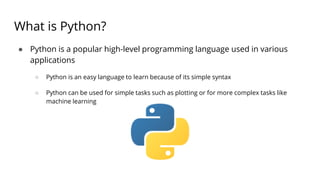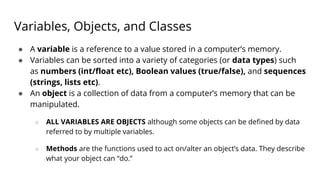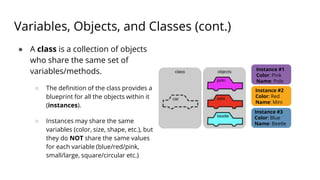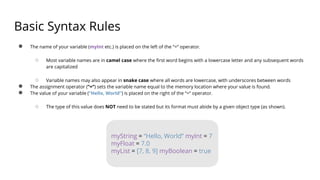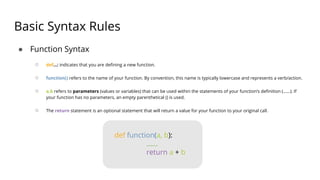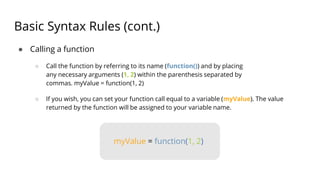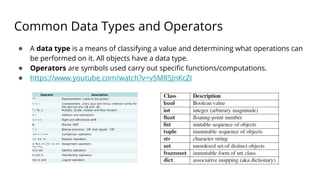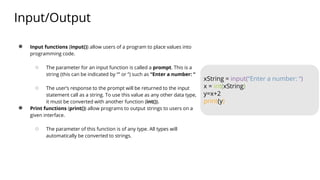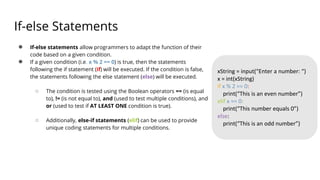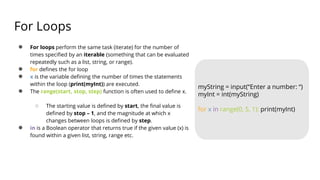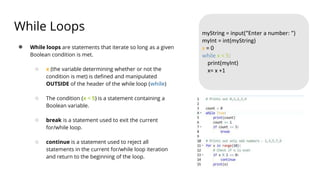1 sur 11
Publicité

### what-is-python-presentation.pptx

1. What is Python? ● Python is a popular high-level programming language used in various applications ○ Python is an easy language to learn because of its simple syntax ○ Python can be used for simple tasks such as plotting or for more complex tasks like machine learning
2. Variables, Objects, and Classes ● A variable is a reference to a value stored in a computer’s memory. ● Variables can be sorted into a variety of categories (or data types) such as numbers (int/float etc), Boolean values (true/false), and sequences (strings, lists etc). ● An object is a collection of data from a computer’s memory that can be manipulated. ○ ALL VARIABLES ARE OBJECTS although some objects can be defined by data referred to by multiple variables. ○ Methods are the functions used to act on/alter an object’s data. They describe what your object can “do.”
3. Variables, Objects, and Classes (cont.) ● A class is a collection of objects who share the same set of variables/methods. ○ The definition of the class provides a blueprint for all the objects within it (instances). ○ Instances may share the same variables (color, size, shape, etc.), but they do NOT share the same values for each variable (blue/red/pink, small/large, square/circular etc.) Instance #1 Color: Pink Name: Polo Instance #2 Color: Red Name: Mini Instance #3 Color: Blue Name: Beetle
4. Basic Syntax Rules ● The name of your variable (myInt etc.) is placed on the left of the “=“ operator. ○ Most variable names are in camel case where the first word begins with a lowercase letter and any subsequent words are capitalized ○ Variable names may also appear in snake case where all words are lowercase, with underscores between words ● The assignment operator (“=“) sets the variable name equal to the memory location where your value is found. ● The value of your variable (“Hello, World”) is placed on the right of the “=“ operator. ○ The type of this value does NOT need to be stated but its format must abide by a given object type (as shown). myString = “Hello, World” myInt = 7 myFloat = 7.0 myList = [7, 8, 9] myBoolean = true
5. Basic Syntax Rules ● Function Syntax ○ def...: indicates that you are defining a new function. ○ function() refers to the name of your function. By convention, this name is typically lowercase and represents a verb/action. ○ a,b refers to parameters (values or variables) that can be used within the statements of your function’s definition (......). If your function has no parameters, an empty parenthetical () is used. ○ The return statement is an optional statement that will return a value for your function to your original call. def function(a, b): ...... return a + b
6. Basic Syntax Rules (cont.) ● Calling a function ○ Call the function by referring to its name (function()) and by placing any necessary arguments (1, 2) within the parenthesis separated by commas. myValue = function(1, 2) ○ If you wish, you can set your function call equal to a variable (myValue). The value returned by the function will be assigned to your variable name. myValue = function(1, 2)
7. Common Data Types and Operators ● A data type is a means of classifying a value and determining what operations can be performed on it. All objects have a data type. ● Operators are symbols used carry out specific functions/computations. ● https://www.youtube.com/watch?v=v5MR5JnKcZI
8. Input/Output ● Input functions (input()) allow users of a program to place values into programming code. ○ The parameter for an input function is called a prompt. This is a string (this can be indicated by “” or ‘’) such as “Enter a number: “ ○ The user’s response to the prompt will be returned to the input statement call as a string. To use this value as any other data type, it must be converted with another function (int()). ● Print functions (print()) allow programs to output strings to users on a given interface. ○ The parameter of this function is of any type. All types will automatically be converted to strings. xString = input(“Enter a number: “) x = int(xString) y=x+2 print(y)
9. If-else Statements ● If-else statements allow programmers to adapt the function of their code based on a given condition. ● If a given condition (i.e. x % 2 == 0) is true, then the statements following the if statement (if) will be executed. If the condition is false, the statements following the else statement (else) will be executed. ○ The condition is tested using the Boolean operators == (is equal to), != (is not equal to), and (used to test multiple conditions), and or (used to test if AT LEAST ONE condition is true). ○ Additionally, else-if statements (elif) can be used to provide unique coding statements for multiple conditions. xString = input(“Enter a number: “) x = int(xString) if x % 2 == 0: print(“This is an even number”) elif x == 0: print(“This number equals 0”) else: print(“This is an odd number”)
10. For Loops ● For loops perform the same task (iterate) for the number of times specified by an iterable (something that can be evaluated repeatedly such as a list, string, or range). ● for defines the for loop ● x is the variable defining the number of times the statements within the loop (print(myInt)) are executed. ● The range(start, stop, step) function is often used to define x. ○ The starting value is defined by start, the final value is defined by stop – 1, and the magnitude at which x changes between loops is defined by step. ● in is a Boolean operator that returns true if the given value (x) is found within a given list, string, range etc. myString = input(“Enter a number: “) myInt = int(myString) for x in range(0, 5, 1): print(myInt)
11. While Loops ● While loops are statements that iterate so long as a given Boolean condition is met. ○ x (the variable determining whether or not the condition is met) is defined and manipulated OUTSIDE of the header of the while loop (while) ○ The condition (x < 5) is a statement containing a Boolean variable. ○ break is a statement used to exit the current for/while loop. ○ continue is a statement used to reject all statements in the current for/while loop iteration and return to the beginning of the loop. myString = input(“Enter a number: “) myInt = int(myString) x = 0 while x < 5: print(myInt) x= x +1
Publicité